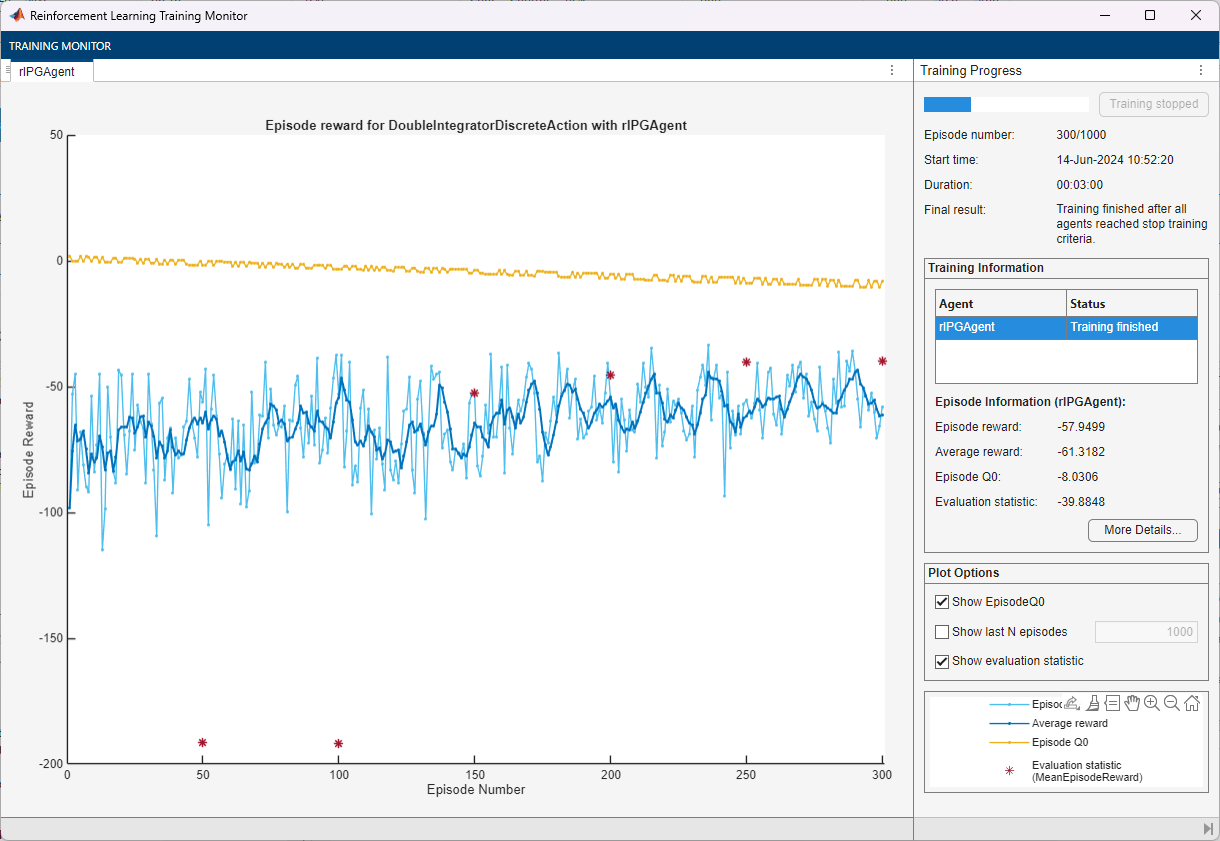Main Content

# Train PG Agent with Baseline to Control Double Integrator System

This example shows how to train a policy gradient (PG) agent with baseline to control a second-order dynamic system modeled in MATLAB®.

For more information on the basic PG agent with no baseline, see the example Train PG Agent to Balance Cart-Pole System.

### Double Integrator MATLAB Environment

The reinforcement learning environment for this example is a second-order double integrator system with a gain. The training goal is to control the position of a mass in the second-order system by applying a force input.For this environment:

• The mass starts at an initial position between –2 and 2 units.

• The force action signal from the agent to the environment is from –2 to 2 N.

• The observations from the environment are the position and velocity of the mass.

• The episode terminates if the mass moves more than 5 m from the original position or if $|\mathit{x}|<0.01$.

• The reward ${\mathit{r}}_{\mathit{t}}$, provided at every time step, is a discretization of $\mathit{r}\left(\mathit{t}\right)$:

`$\mathit{r}\left(\mathit{t}\right)=-\left({\mathit{x}\left(\mathit{t}\right)}^{\prime }\text{\hspace{0.17em}}\mathit{Q}\text{\hspace{0.17em}}\mathit{x}\left(\mathit{t}\right)+{\mathit{u}\left(\mathit{t}\right)}^{\prime }\text{\hspace{0.17em}}\mathit{R}\text{\hspace{0.17em}}\mathit{u}\left(\mathit{t}\right)\right)$`

Here:

• $\mathit{x}$ is the state vector of the mass.

• $\mathit{u}$ is the force applied to the mass.

• $\mathit{Q}$ is the weights on the control performance; $\mathit{Q}=\left[10\text{\hspace{0.17em}}0;0\text{\hspace{0.17em}}1\right]$.

• $\mathit{R}$ is the weight on the control effort; $\mathit{R}=0.01$.

For more information on this model, see Load Predefined Control System Environments.

### Create Double Integrator MATLAB Environment Interface

Create a predefined environment interface for the pendulum.

`env = rlPredefinedEnv("DoubleIntegrator-Discrete")`
```env = DoubleIntegratorDiscreteAction with properties: Gain: 1 Ts: 0.1000 MaxDistance: 5 GoalThreshold: 0.0100 Q: [2x2 double] R: 0.0100 MaxForce: 2 State: [2x1 double] ```

The interface has a discrete action space where the agent can apply one of three possible force values to the mass: -2, 0, or 2 N.

Obtain the observation and action information from the environment interface.

```obsInfo = getObservationInfo(env); numObservations = obsInfo.Dimension(1); actInfo = getActionInfo(env); numActions = numel(actInfo.Elements);```

Fix the random generator seed for reproducibility.

`rng(0)`

### Create PG Agent Actor

A PG agent decides which action to take, given observations, using an actor representation. To create the actor, first create a deep neural network with one input (the observation) and one output (the action). For more information on creating a deep neural network value function representation, see Create Policy and Value Function Representations.

```actorNetwork = [ featureInputLayer(numObservations,'Normalization','none','Name','state') fullyConnectedLayer(numActions,'Name','action','BiasLearnRateFactor',0)];```

Specify options for the actor representation using `rlRepresentationOptions`.

`actorOpts = rlRepresentationOptions('LearnRate',5e-3,'GradientThreshold',1);`

Create the actor representation using the specified deep neural network and options. You must also specify the action and observation information for the critic, which you obtained from the environment interface. For more information, see `rlStochasticActorRepresentation`.

`actor = rlStochasticActorRepresentation(actorNetwork,obsInfo,actInfo,'Observation',{'state'},actorOpts);`

### Create PG Agent Baseline

A baseline that varies with state can reduce the variance of the expected value of the update and thus improve the speed of learning for a PG agent. A possible choice for the baseline is an estimate of the state value function .

In this case, the baseline representation is a deep neural network with one input (the state) and one output (the state value).

Construct the baseline in a similar manner to the actor.

```baselineNetwork = [ featureInputLayer(numObservations,'Normalization','none','Name','state') fullyConnectedLayer(8,'Name','BaselineFC') reluLayer('Name','CriticRelu1') fullyConnectedLayer(1,'Name','BaselineFC2','BiasLearnRateFactor',0)]; baselineOpts = rlRepresentationOptions('LearnRate',5e-3,'GradientThreshold',1); baseline = rlValueRepresentation(baselineNetwork,obsInfo,'Observation',{'state'},baselineOpts);```

To create the PG agent with baseline, specify the PG agent options using `rlPGAgentOptions` and set the `UseBaseline` option set to `true`.

```agentOpts = rlPGAgentOptions(... 'UseBaseline',true, ... 'DiscountFactor',0.99);```

Then create the agent using the specified actor representation, baseline representation, and agent options. For more information, see `rlPGAgent`.

`agent = rlPGAgent(actor,baseline,agentOpts);`

### Train Agent

To train the agent, first specify the training options. For this example, use the following options.

• Run at most 1000 episodes, with each episode lasting at most 200 time steps.

• Display the training progress in the Episode Manager dialog box (set the `Plots` option) and disable the command line display (set the `Verbose` option).

• Stop training when the agent receives a moving average cumulative reward greater than –45. At this point, the agent can control the position of the mass using minimal control effort.

For more information, see `rlTrainingOptions`.

```trainOpts = rlTrainingOptions(... 'MaxEpisodes',1000, ... 'MaxStepsPerEpisode',200, ... 'Verbose',false, ... 'Plots','training-progress',... 'StopTrainingCriteria','AverageReward',... 'StopTrainingValue',-43);```

You can visualize the double integrator system using the `plot` function during training or simulation.

`plot(env)`Train the agent using the `train` function. Training this agent is a computationally intensive process that takes several minutes to complete. To save time while running this example, load a pretrained agent by setting `doTraining` to `false`. To train the agent yourself, set `doTraining` to `true`.

```doTraining = false; if doTraining % Train the agent. trainingStats = train(agent,env,trainOpts); else % Load the pretrained parameters for the example. load('DoubleIntegPGBaseline.mat'); end```### Simulate PG Agent

To validate the performance of the trained agent, simulate it within the double integrator environment. For more information on agent simulation, see `rlSimulationOptions` and `sim`.

```simOptions = rlSimulationOptions('MaxSteps',500); experience = sim(env,agent,simOptions);````totalReward = sum(experience.Reward)`
```totalReward = -43.0392 ```

### References

 Sutton, Richard S., and Andrew G. Barto. Reinforcement Learning: An Introduction. Second edition. Adaptive Computation and Machine Learning Series. Cambridge, MA: The MIT Press, 2018.

Download ebook